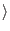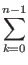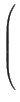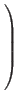Next: Probability Interpretation Up: The Quantum Computer Previous: The Quantum qubit   Contents

## The Quantum Memory Register

Up till now we have considered a two state quantum system, specifically our spin-1/2 particle. However a quantum system is by no means constrained to be a two state system. Much of the above discussion for a 2 state quantum system is applicable to a general n state quantum system.

In an n state system our Hilbert Space has n perpendicular axes, or eigenstates, which represent the possible states that the system can be measured in. As with the two state system, when we measure our n state quantum system, we will always find it to be in exactly one of the n states, and not a superposition of the n states. The system is still allowed to exist in any superposition of the n states while it is not being measured.

Mathematically if two state quantum system with coordinate axes x0, x1 can be fully described by:

| X= w0*| x0+ w1*| x1(w0, w1)

Then an n state quantum system with coordinate axes x0, x1,..., xn-1 can be fully described by:

| X=wk*| xkIn general a quantum system with n base states can be represented by the n complex numbers w0 to wn-1. When this is done the state may be written as:

| X=Where it is understood that wk refers to the complex weighting factor for the k'th eigenstate.

Using this information we can construct a quantum memory register out of the qubits described in the previous section. We may store any number n in our quantum memory register as long as we have enough qubits, just as we may store any number n in a classical register as long as we have enough classical bits to represent that number. The state of the quantum register with n states is give by the formula above. Note that in general a quantum register composed of n qubits requires 2n complex numbers to completely describe its state. A n qubit register can be measured to be in one of 2n states, and each state requires one complex number to represent the projection of that total state onto that state. In contrast a classical register composed of n bits requires only n integers to fully describe its state.

This means that one can store an exponential amount of information in a quantum register relative to the size of that register. Here we see some of the first hints that a quantum computer could be exponentially more efficient than a classical computer in some respects. Recall that from our discussion of complexity that problems which can be solved in polynomial time are generally thought of as being tractable, and that problems which can be solved in exponential time are thought of as intractable. If a quantum computer can be is exponentially more efficient than a classical computer for some algorithms, then some problems currently intractable may become tractable! This is a large part of the motivation for the study of quantum computing.Next: Probability Interpretation Up: The Quantum Computer Previous: The Quantum qubit   Contents
Matthew Hayward - Quantum Computing and Shor's Algorithm GitHub Repository